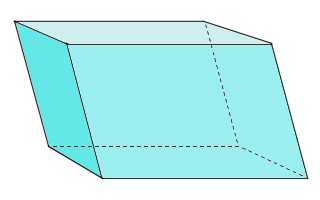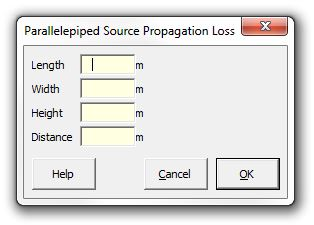﻿ Parallelepiped### Strutt Help

Parallelepiped Source Propagation Loss    1/1, 1/3

Strutt|Propagation|Parallelepiped provides the correction for sound power radiated over a parallelepiped, given the length, width, height and distance in metres from the source.This is implemented as:

x = 10log_10(S), where

S = 4[(1/2 A + r)(1/2 B + r) + (1/2 B + r)(C + r) + (C + r)(1/2 A + r)]

Note:

• This calculation assumes that the source is located on a reflective plane.
• For the vast majority of situations, the conformal area calculation is more appropriate. However, some ISO standards require the use of this parallelepiped calculation.